JMSLTM Numerical Library 7.2.0
com.imsl.stat

Class SelectionRegression

• All Implemented Interfaces:
Serializable, Cloneable

public class SelectionRegression
extends Object
implements Serializable, Cloneable
Selects the best multiple linear regression models.

Class SelectionRegression finds the best subset regressions for a regression problem with three or more independent variables. Typically, the intercept is forced into all models and is not a candidate variable. In this case, a sum of squares and crossproducts matrix for the independent and dependent variables corrected for the mean is computed internally. Optionally, SelectionRegression supports user-calculated sum-of-squares and crossproducts matrices; see the description of the compute method.

"Best" is defined by using one of the following three criteria:

•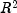(in percent)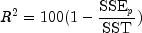•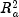(adjusted)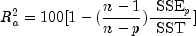Note that maximizing theis equivalent to minimizing the residual mean squared error: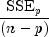• Mallow's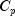statistic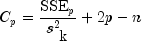Here, n is equal to the sum of the frequencies (or the number of rows in x if frequencies are not specified in the compute method), and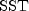is the total sum of squares. k is the number of candidate or independent variables, represented as the nCandidate argument in the SelectionRegression constructor.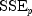is the error sum of squares in a model containing p regression parameters including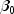(or p - 1 of the k candidate variables). Variable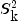is the error mean square from the model with all k variables in the model. Hocking (1972) and Draper and Smith (1981, pp. 296-302) discuss these criteria.

Class SelectionRegression is based on the algorithm of Furnival and Wilson (1974). This algorithm finds the maximum number of good saved candidate regressions for each possible subset size. For more details, see method setMaximumGoodSaved(int). These regressions are used to identify a set of best regressions. In large problems, many regressions are not computed. They may be rejected without computation based on results for other subsets; this yields an efficient technique for considering all possible regressions.

There are cases when the user may want to input the variance-covariance matrix rather than allow it to be calculated. This can be accomplished using the appropriate compute method. Three situations in which the user may want to do this are as follows:

1. The intercept is not in the model. A raw (uncorrected) sum of squares and crossproducts matrix for the independent and dependent variables is required. Argument nObservations must be set to 1 greater than the number of observations. Form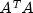, where A = [A, Y], to compute the raw sum of squares and crossproducts matrix.
2. An intercept is a candidate variable. A raw (uncorrected) sum of squares and crossproducts matrix for the constant regressor (= 1.0), independent, and dependent variables is required for cov . In this case, cov contains one additional row and column corresponding to the constant regressor. This row and column contain the sum of squares and crossproducts of the constant regressor with the independent and dependent variables. The remaining elements in cov are the same as in the previous case. Argument nObservations must be set to 1 greater than the number of observations.
3. There are m variables that must be forced into the models. A sum of squares and crossproducts matrix adjusted for the m variables is required (calculated by regressing the candidate variables on the variables to be forced into the model). Argument nObservations must be set to m less than the number of observations.

Programming Notes

SelectionRegression can save considerable CPU time over explicitly computing all possible regressions. However, the function has some limitations that can cause unexpected results for users who are unaware of the limitations of the software.
1. For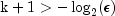, whereis the largest relative spacing for double precision, some results can be incorrect. This limitation arises because the possible models indicated (the model numbers 1, 2, ..., 2k) are stored as floating-point values; for sufficiently large k, the model numbers cannot be stored exactly. On many computers, this means SelectionRegression (for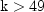) can produce incorrect results.
2. SelectionRegression eliminates some subsets of candidate variables by obtaining lower bounds on the error sum of squares from fitting larger models. First, the full model containing all independent variables is fit sequentially using a forward stepwise procedure in which one variable enters the model at a time, and criterion values and model numbers for all the candidate variables that can enter at each step are stored. If linearly dependent variables are removed from the full model, a "VariablesDeleted" warning is issued. In this case, some submodels that contain variables removed from the full model because of linear dependency can be overlooked if they have not already been identified during the initial forward stepwise procedure. If this warning is issued and you want the variables that were removed from the full model to be considered in smaller models, you can rerun the program with a set of linearly independent variables.
Example 1, Example 2, Serialized Form
• Field Summary

Fields
Modifier and Type Field and Description
Indicates(adjusted) criterion regression.
static int MALLOWS_CP_CRITERION
Indicates Mallow'scriterion regression.
static int R_SQUARED_CRITERION
Indicatescriterion regression.
• Constructor Summary

Constructors
Constructor and Description
SelectionRegression(int nCandidate)
Constructs a new SelectionRegression object.
• Method Summary

Methods
Modifier and Type Method and Description
void compute(double[][] x, double[] y)
Computes the best multiple linear regression models.
void compute(double[][] x, double[] y, double[] weights)
Computes the best weighted multiple linear regression models.
void compute(double[][] x, double[] y, double[] weights, double[] frequencies)
Computes the best weighted multiple linear regression models using frequencies for each observation.
void compute(double[][] cov, int nObservations)
Computes the best multiple linear regression models using a user-supplied covariance matrix.
int getCriterionOption()
Returns the criterion option used to calculate the regression estimates.
SelectionRegression.Statistics getStatistics()
Returns a new Statistics object.
void setCriterionOption(int criterionOption)
Sets the Criterion to be used.
void setMaximumBestFound(int maxFound)
Sets the maximum number of best regressions to be found.
void setMaximumGoodSaved(int maxSaved)
Sets the maximum number of good regressions for each subset size saved.
void setMaximumSubsetSize(int maxSubset)
Sets the maximum subset size ifcriterion is used.
• Field Detail

Indicates(adjusted) criterion regression.
Constant Field Values
• MALLOWS_CP_CRITERION

public static final int MALLOWS_CP_CRITERION
Indicates Mallow'scriterion regression.
Constant Field Values
• R_SQUARED_CRITERION

public static final int R_SQUARED_CRITERION
Indicatescriterion regression.
Constant Field Values
• Constructor Detail

• SelectionRegression

public SelectionRegression(int nCandidate)
Constructs a new SelectionRegression object.
Parameters:
nCandidate - An int containing the number of candidate variables (independent variables). nCandidate must be greater than 2.
• Method Detail

• compute

public void compute(double[][] x,
double[] y)
throws SelectionRegression.NoVariablesException,
com.imsl.stat.Covariances.TooManyObsDeletedException,
com.imsl.stat.Covariances.MoreObsDelThanEnteredException,
com.imsl.stat.Covariances.DiffObsDeletedException
Computes the best multiple linear regression models.
Parameters:
x - A double matrix containing the observations of the candidate (independent) variables. The number of columns in x must be equal to the number of variables set in the constructor.
y - A double array containing the observations of the dependent variable.
Throws:
SelectionRegression.NoVariablesException - if no variables can enter any model
com.imsl.stat.Covariances.TooManyObsDeletedException - more observations have been deleted than were originally entered
com.imsl.stat.Covariances.MoreObsDelThanEnteredException - more observations are being deleted from the output covariance matrix than were originally entered
com.imsl.stat.Covariances.DiffObsDeletedException - different observations are being deleted from return matrix than were originally entered
• compute

public void compute(double[][] x,
double[] y,
double[] weights)
throws SelectionRegression.NoVariablesException,
Covariances.NonnegativeWeightException,
com.imsl.stat.Covariances.TooManyObsDeletedException,
com.imsl.stat.Covariances.MoreObsDelThanEnteredException,
com.imsl.stat.Covariances.DiffObsDeletedException
Computes the best weighted multiple linear regression models.
Parameters:
x - A double matrix containing the observations of the candidate (independent) variables. The number of columns in x must be equal to the number of variables set in the constructor.
y - A double array containing the observations of the dependent variable.
weights - A double array containing the weight for each of the observations.
Throws:
SelectionRegression.NoVariablesException - if no variables can enter any model
Covariances.NonnegativeWeightException - weights must be nonnegative
com.imsl.stat.Covariances.TooManyObsDeletedException - more observations have been deleted than were originally entered
com.imsl.stat.Covariances.MoreObsDelThanEnteredException - more observations are being deleted from the output covariance matrix than were originally entered
com.imsl.stat.Covariances.DiffObsDeletedException - different observations are being deleted from return matrix than were originally entered
• compute

public void compute(double[][] x,
double[] y,
double[] weights,
double[] frequencies)
throws SelectionRegression.NoVariablesException,
Covariances.NonnegativeFreqException,
Covariances.NonnegativeWeightException,
com.imsl.stat.Covariances.TooManyObsDeletedException,
com.imsl.stat.Covariances.MoreObsDelThanEnteredException,
com.imsl.stat.Covariances.DiffObsDeletedException
Computes the best weighted multiple linear regression models using frequencies for each observation.
Parameters:
x - A double matrix containing the observations of the candidate (independent) variables. The number of columns in x must be equal to the number of variables set in the constructor.
y - A double array containing the observations of the dependent variable.
weights - A double array containing the weight for each of the observations.
frequencies - A double array containing the frequency for each of the observations of x.
Throws:
SelectionRegression.NoVariablesException - if no variables can enter any model
Covariances.NonnegativeFreqException - frequencies must be nonnegative
Covariances.NonnegativeWeightException - weights must be nonnegative
com.imsl.stat.Covariances.TooManyObsDeletedException - more observations have been deleted than were originally entered
com.imsl.stat.Covariances.MoreObsDelThanEnteredException - more observations are being deleted from the output covariance matrix than were originally entered
com.imsl.stat.Covariances.DiffObsDeletedException - different observations are being deleted from return matrix than were originally entered
• compute

public void compute(double[][] cov,
int nObservations)
throws SelectionRegression.NoVariablesException
Computes the best multiple linear regression models using a user-supplied covariance matrix.
Parameters:
cov - A double matrix containing a variance-covariance or sum of squares and crossproducts matrix, in which the last column must correspond to the dependent variable. cov can be computed using the Covariances class.
nObservations - An int containing the number of observations used to compute cov.
Throws:
SelectionRegression.NoVariablesException - if no variables can enter any model
• getStatistics

public SelectionRegression.Statistics getStatistics()
Returns a new Statistics object.
Returns:
A Statistics object containing the Coefficient statistics.
• setCriterionOption

public void setCriterionOption(int criterionOption)
Sets the Criterion to be used. By default for all criteria, subset size 1,2, ..., k = nCandidate are considered. However, forthe maximum number of subsets can be restricted to maxSubset in the setMaximumSubsetSize(int) method.

 Criterion Option Description R_SQUARED_CRITERION For, subset sizes 1, 2, ..., maxSubset are examined. This is the default with maxSubset = nCandidate. ADJUSTED_R_SQUARED_CRITERION For Adjusted, subset sizes 1, 2, ..., nCandidate are examined. MALLOWS_CP_CRITERION For Mallow'sSubset sizes 1, 2, ..., nCandidate are examined.

Parameters:
criterionOption - An int containing the criterion option used for the best subset regression selection.
• setMaximumBestFound

public void setMaximumBestFound(int maxFound)
Sets the maximum number of best regressions to be found.

If thecriterion option is selected, the maxFound best regressions for each subset size examined are reported. If the adjustedor Mallow'scriteria are selected, the maxFound among all possible regressions are found.

Parameters:
maxFound - An int containing the maximum number of best regressions to be reported. Default: maxFound = 1.
• setMaximumGoodSaved

public void setMaximumGoodSaved(int maxSaved)
Sets the maximum number of good regressions for each subset size saved.

Argument maxSaved must be greater than or equal to maxFound. Normally, maxSaved should be less than or equal to 10. It should never need be larger than maxSubset, the maximum number of subsets for any subset size. Computing time required is inversely related to maxSaved.

Parameters:
maxSaved - An int containing the maximum number of good regressions saved for each subset size. Default: maxSaved = maximum(10, maxSubset).
• setMaximumSubsetSize

public void setMaximumSubsetSize(int maxSubset)
Sets the maximum subset size ifcriterion is used.
Parameters:
maxSubset - An int containing the maximum subset size whencriterion is used. Default: maxSubset = nCandidate.